## Activation Energy

Activation energy is the energy required for a reaction to occur, and determines its rate.

### Learning Objectives

Discuss the concept of activation energy

### Key Takeaways

#### Key Points

• Reactions require an input of energy to initiate the reaction; this is called the activation energy (EA).
• Activation energy is the amount of energy required to reach the transition state.
• The source of the activation energy needed to push reactions forward is typically heat energy from the surroundings.
• For cellular reactions to occur fast enough over short time scales, their activation energies are lowered by molecules called catalysts.
• Enzymes are catalysts.

#### Key Terms

• activation energy: The minimum energy required for a reaction to occur.
• catalysis: The increase in the rate of a chemical reaction by lowering its activation energy.
• transition state: An intermediate state during a chemical reaction that has a higher energy than the reactants or the products.

Many chemical reactions, and almost all biochemical reactions do not occur spontaneously and must have an initial input of energy (called the activation energy) to get started. Activation energy must be considered when analyzing both endergonic and exergonic reactions. Exergonic reactions have a net release of energy, but they still require a small amount of energy input before they can proceed with their energy-releasing steps. This small amount of energy input necessary for all chemical reactions to occur is called the activation energy (or free energy of activation) and is abbreviated EA.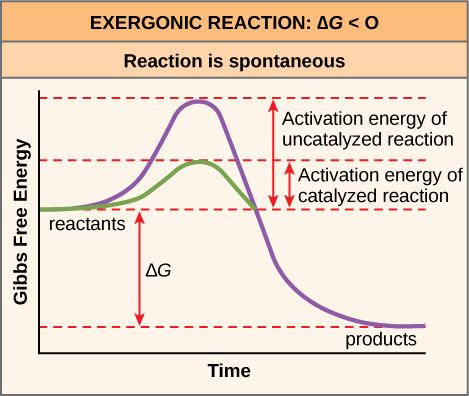Activation energy: Activation energy is the energy required for a reaction to proceed; it is lower if the reaction is catalyzed. The horizontal axis of this diagram describes the sequence of events in time.

### Activation Energy in Chemical Reactions

Why would an energy-releasing, negative ∆G reaction actually require some energy to proceed? The reason lies in the steps that take place during a chemical reaction. During chemical reactions, certain chemical bonds are broken and new ones are formed. For example, when a glucose molecule is broken down, bonds between the carbon atoms of the molecule are broken. Since these are energy-storing bonds, they release energy when broken. However, to get them into a state that allows the bonds to break, the molecule must be somewhat contorted. A small energy input is required to achieve this contorted state, which is called the transition state: it is a high-energy, unstable state. For this reason, reactant molecules don’t last long in their transition state, but very quickly proceed to the next steps of the chemical reaction.

Cells will at times couple an exergonic reaction $(\Delta \text{G}\lt0)$ with endergonic reactions $(\Delta \text{G}\gt0)$, allowing them to proceed. This spontaneous shift from one reaction to another is called energy coupling. The free energy released from the exergonic reaction is absorbed by the endergonic reaction. One example of energy coupling using ATP involves a transmembrane ion pump that is extremely important for cellular function.

### Free Energy Diagrams

Free energy diagrams illustrate the energy profiles for a given reaction. Whether the reaction is exergonic (ΔG<0) or endergonic (ΔG>0) determines whether the products in the diagram will exist at a lower or higher energy state than the reactants. However, the measure of the activation energy is independent of the reaction’s ΔG. In other words, at a given temperature, the activation energy depends on the nature of the chemical transformation that takes place, but not on the relative energy state of the reactants and products.

Although the image above discusses the concept of activation energy within the context of the exergonic forward reaction, the same principles apply to the reverse reaction, which must be endergonic. Notice that the activation energy for the reverse reaction is larger than for the forward reaction.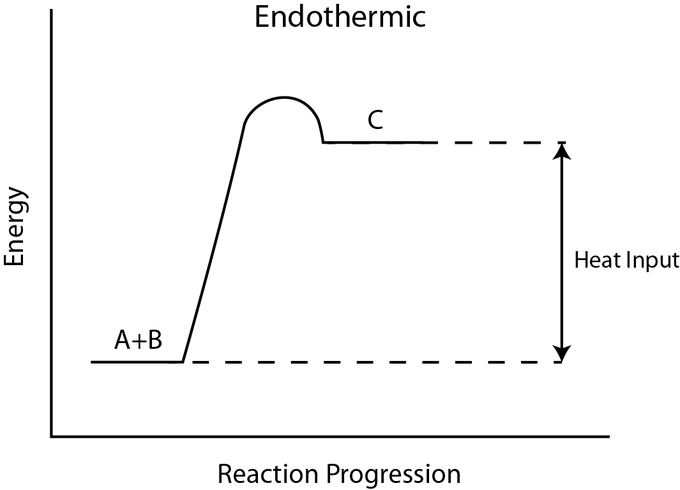Activation energy in an endergonic reaction: In this endergonic reaction, activation energy is still required to transform the reactants A + B into the product C. This figure implies that the activation energy is in the form of heat energy.

### Heat Energy

The source of the activation energy needed to push reactions forward is typically heat energy from the surroundings. Heat energy (the total bond energy of reactants or products in a chemical reaction) speeds up the motion of molecules, increasing the frequency and force with which they collide. It also moves atoms and bonds within the molecule slightly, helping them reach their transition state. For this reason, heating up a system will cause chemical reactants within that system to react more frequently. Increasing the pressure on a system has the same effect. Once reactants have absorbed enough heat energy from their surroundings to reach the transition state, the reaction will proceed.

The activation energy of a particular reaction determines the rate at which it will proceed. The higher the activation energy, the slower the chemical reaction will be. The example of iron rusting illustrates an inherently slow reaction. This reaction occurs slowly over time because of its high EA. Additionally, the burning of many fuels, which is strongly exergonic, will take place at a negligible rate unless their activation energy is overcome by sufficient heat from a spark. Once they begin to burn, however, the chemical reactions release enough heat to continue the burning process, supplying the activation energy for surrounding fuel molecules.

Like these reactions outside of cells, the activation energy for most cellular reactions is too high for heat energy to overcome at efficient rates. In other words, in order for important cellular reactions to occur at significant rates (number of reactions per unit time), their activation energies must be lowered; this is referred to as catalysis. This is a very good thing as far as living cells are concerned. Important macromolecules, such as proteins, DNA, and RNA, store considerable energy, and their breakdown is exergonic. If cellular temperatures alone provided enough heat energy for these exergonic reactions to overcome their activation barriers, the essential components of a cell would disintegrate.

### The Arrhenius Equation

The Arrhenius equations relates the rate of a chemical reaction to the magnitude of the activation energy:

$\text{k}=\text{Ae}^{\text{E}_\text{a}/\text{RT}}$

where

• k is the reaction rate coefficient or constant
• A is the frequency factor of the reaction. It is determined experimentally.
• R is the Universal Gas constant
• T is the temperature in Kelvin

## The Collision Theory

Collision theory provides a qualitative explanation of chemical reactions and the rates at which they occur, appealing to the principle that molecules must collide to react.

### Learning Objectives

Discuss the role of activation energy, collisions, and molecular orientation in collision theory

### Key Takeaways

#### Key Points

• Molecules must collide in order to react.
• In order to effectively initiate a reaction, collisions must be sufficiently energetic ( kinetic energy ) to break chemical bonds; this energy is known as the activation energy.
• As the temperature rises, molecules move faster and collide more vigorously, greatly increasing the likelihood of bond breakage upon collision.

#### Key Terms

• activation energy: The minimum energy with which reactants must collide in order for a reaction to occur.

Collision Theory provides a qualitative explanation of chemical reactions and the rates at which they occur. A basic principal of collision theory is that, in order to react, molecules must collide. This fundamental rule guides any analysis of an ordinary reaction mechanism.

Consider the elementary bimolecular reaction: $\text{A} + \text{B} \rightarrow \text{products}$

If the two molecules A and B are to react, they must come into contact with sufficient force so that chemical bonds break. We call such an encounter a collision. If both A and B are gases, the frequency of collisions between A and B will be proportional to the concentration of each gas. If we double the concentration of A, the frequency of A-B collisions will double, and doubling the concentration of B will have the same effect. Therefore, according to collision theory, the rate at which molecules collide will have an impact on the overall reaction rate.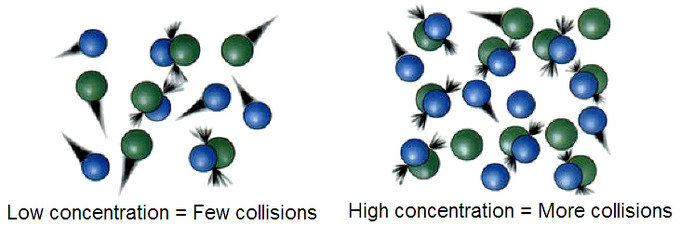Molecular collisions: The more molecules present, the more collisions will happen.

### Activation Energy and Temperature

When two billiard balls collide, they simply bounce off of one other. This is also the most likely outcome when two molecules, A and B, come into contact: they bounce off one another, completely unchanged and unaffected. In order for a collision to be successful by resulting in a chemical reaction, A and B must collide with sufficient energy to break chemical bonds. This is because in any chemical reaction, chemical bonds in the reactants are broken, and new bonds in the products are formed. Therefore, in order to effectively initiate a reaction, the reactants must be moving fast enough (with enough kinetic energy) so that they collide with sufficient force for bonds to break. This minimum energy with which molecules must be moving in order for a collision to result in a chemical reaction is known as the activation energy.

As we know from the kinetic theory of gases, the kinetic energy of a gas is directly proportional to temperature. As temperature increases, molecules gain energy and move faster and faster. Therefore, the greater the temperature, the higher the probability that molecules will be moving with the necessary activation energy for a reaction to occur upon collision.

### Molecular Orientation and Effective Collisions

Even if two molecules collide with sufficient activation energy, there is no guarantee that the collision will be successful. In fact, the collision theory says that not every collision is successful, even if molecules are moving with enough energy. The reason for this is because molecules also need to collide with the right orientation, so that the proper atoms line up with one another, and bonds can break and re-form in the necessary fashion. For example, in the gas- phase reaction of dinitrogen oxide with nitric oxide, the oxygen end of N2O must hit the nitrogen end of NO; if either molecule is not lined up correctly, no reaction will occur upon their collision, regardless of how much energy they have. However, because molecules in the liquid and gas phase are in constant, random motion, there is always the probability that two molecules will collide in just the right way for them to react.

Of course, the more critical this orientational requirement is, like it is for larger or more complex molecules, the fewer collisions there will be that will be effective. An effective collision is defined as one in which molecules collide with sufficient energy and proper orientation, so that a reaction occurs.

### Conclusion

According to the collision theory, the following criteria must be met in order for a chemical reaction to occur:

1. Molecules must collide with sufficient energy, known as the activation energy, so that chemical bonds can break.
2. Molecules must collide with the proper orientation.
3. A collision that meets these two criteria, and that results in a chemical reaction, is known as a successful collision or an effective collision.

Collision theory explanation: Collision theory provides an explanation for how particles interact to cause a reaction and the formation of new products.

## Factors that Affect Reaction Rate

The rate of a chemical reaction depends on factors that affect whether reactants can collide with sufficient energy for reaction to occur.

### Learning Objectives

Explain how concentration, surface area, pressure, temperature, and the addition of catalysts affect reaction rate

### Key Takeaways

#### Key Points

• When the concentrations of the reactants are raised, the reaction proceeds more quickly. This is due to an increase in the number of molecules that have the minimum required energy. For gases, increasing pressure has the same effect as increasing concentration.
• When solids and liquids react, increasing the surface area of the solid will increase the reaction rate. A decrease in particle size causes an increase in the solid’s total surface area.
• Raising the reaction temperature by 10 °C can double or triple the reaction rate. This is due to an increase in the number of particles that have the minimum energy required. The reaction rate decreases with a decrease in temperature.
• Catalysts can lower the activation energy and increase the reaction rate without being consumed in the reaction.
• Differences in the inherent structures of reactants can lead to differences in reaction rates. Molecules joined by stronger bonds will have lower reaction rates than will molecules joined by weaker bonds, due to the increased amount of energy required to break the stronger bonds.

#### Key Terms

• catalyst: A substance that increases the rate of a chemical reaction without being consumed in the process.
• activation energy: The minimum amount of energy that molecules must have in order for a reaction to occur upon collision.

### Reactant Concentrations

Raising the concentrations of reactants makes the reaction happen at a faster rate. For a chemical reaction to occur, there must be a certain number of molecules with energies equal to or greater than the activation energy. With an increase in concentration, the number of molecules with the minimum required energy will increase, and therefore the rate of the reaction will increase. For example, if one in a million particles has sufficient activation energy, then out of 100 million particles, only 100 will react. However, if you have 200 million of those particles within the same volume, then 200 of them react. By doubling the concentration, the rate of reaction has doubled as well.

Interactive: Concentration and Reaction Rate: In this model, two atoms can form a bond to make a molecule. Experiment with changing the concentration of the atoms in order to see how this affects the reaction rate (the speed at which the reaction occurs).

### Surface Area

In a reaction between a solid and a liquid, the surface area of the solid will ultimately impact how fast the reaction occurs. This is because the liquid and the solid can bump into each other only at the liquid-solid interface, which is on the surface of the solid. The solid molecules trapped within the body of the solid cannot react. Therefore, increasing the surface area of the solid will expose more solid molecules to the liquid, which allows for a faster reaction.

For example, consider a 6 x 6 x 2 inch brick. The area of the exposed surfaces of the brick is $4(6\times 2)+2(6\times 6)=120\;\text{cm}^2$. When the brick is dismantled into nine smaller cubes, however, each cube has a surface area of $6(2 \times 2) = 24\ \text{cm}^2$, so the total surface area of the nine cubes is $9 \times 24 = 216\ \text{cm}^2$.

This shows that the total exposed surface area will increase when a larger body is divided into smaller pieces. Therefore, since a reaction takes place on the surface of a substance, increasing the surface area should increase the quantity of the substance that is available to react, and will thus increase the rate of the reaction as well.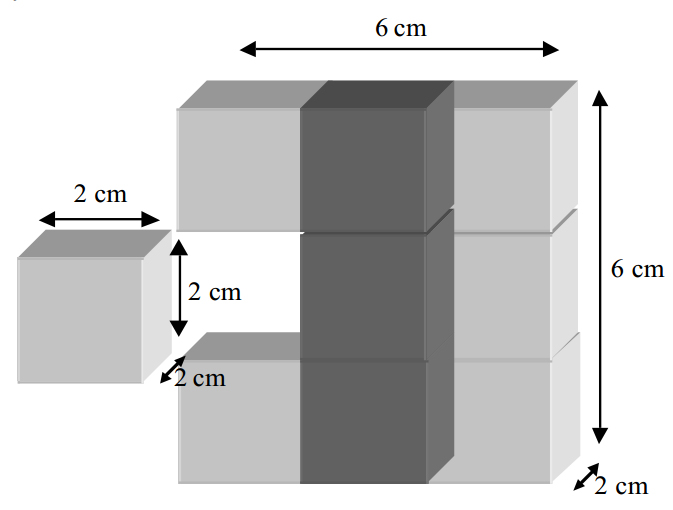Surface areas of smaller molecules versus larger molecules: This picture shows how dismantling a brick into smaller cubes causes an increase in the total surface area.

### Pressure

Increasing the pressure for a reaction involving gases will increase the rate of reaction. As you increase the pressure of a gas, you decrease its volume (PV=nRT; P and V are inversely related), while the number of particles (n) remains unchanged. Therefore, increasing pressure increases the concentration of the gas (n/V), and ensures that the gas molecules collide more frequently. Keep in mind this logic only works for gases, which are highly compressible; changing the pressure for a reaction that involves only solids or liquids has no effect on the reaction rate.

### Temperature

It has been observed experimentally that a rise of 10 °C in temperature usually doubles or triples the speed of a reaction between molecules. The minimum energy needed for a reaction to proceed, known as the activation energy, stays the same with increasing temperature. However, the average increase in particle kinetic energy caused by the absorbed heat means that a greater proportion of the reactant molecules now have the minimum energy necessary to collide and react. An increase in temperature causes a rise in the energy levels of the molecules involved in the reaction, so the rate of the reaction increases. Similarly, the rate of reaction will decrease with a decrease in temperature.

Interactive: Temperature and Reaction Rate: Explore the role of temperature on reaction rate. Note: In this model any heat generated by the reaction itself is removed, keeping the temperature constant in order to isolate the effect of environmental temperature on the rate of reaction.

### Presence or Absence of a Catalyst

Catalysts are substances that increase reaction rate by lowering the activation energy needed for the reaction to occur. A catalyst is not destroyed or changed during a reaction, so it can be used again. For example, at ordinary conditions, H2 and O2 do not combine. However, they do combine in the presence of a small quantity of platinum, which acts as a catalyst, and the reaction then occurs rapidly.

### Nature of the Reactants

Substances differ markedly in the rates at which they undergo chemical change. The differences in reactivity between reactions may be attributed to the different structures of the materials involved; for example, whether the substances are in solution or in the solid state matters. Another factor has to do with the relative bond strengths within the molecules of the reactants. For example, a reaction between molecules with atoms that are bonded by strong covalent bonds will take place at a slower rate than would a reaction between molecules with atoms that are bonded by weak covalent bonds. This is due to the fact that it takes more energy to break the bonds of the strongly bonded molecules.

## The Arrhenius Equation

The Arrhenius equation is a formula that describes the temperature-dependence of a reaction rate.

### Learning Objectives

Explain the Arrhenius equation and the meaning of the variables contained within it

### Key Takeaways

#### Key Points

• The equation relates k, the rate constant for a given chemical reaction, with the temperature, T, the activation energy for the reaction, Ea, the pre-exponential factor A, and the universal gas constant, R.
• High temperature and low activation energy favor larger rate constants, and therefore speed up the reaction.
• The equation is a combination of the concepts of activation energy and the Maxwell-Boltzmann distribution.

#### Key Terms

• Exponential Decay: When a quantity decreases at a rate proportional to its value.

The Arrhenius equation is a simple but remarkably accurate formula for the temperature dependence of the reaction rate constant, and therefore, the rate of a chemical reaction. The equation was first proposed by Svante Arrhenius in 1884. Five years later, in 1889, Dutch chemist J. H. van ‘t Hoff provided physical justification and interpretation for it. The equation combines the concepts of activation energy and the Boltzmann distribution law into one of the most important relationships in physical chemistry:

$\text{k}= \text{Ae}^{-\frac{\text{E}_\text{a}}{\text{RT}}}$

In this equation, k is the rate constant, T is the absolute temperature, Ea is the activation energy, A is the pre-exponential factor, and R is the universal gas constant.

Take a moment to focus on the meaning of this equation, neglecting the A factor for the time being. First, note that this is another form of the exponential decay law. What is “decaying” here is not the concentration of a reactant as a function of time, but the magnitude of the rate constant as a function of the exponent –Ea /RT.

What is the significance of this quantity? If you recall that RT is the average kinetic energy, it will be apparent that the exponent is just the ratio of the activation energy, Ea, to the average kinetic energy. The larger this ratio, the smaller the rate, which is why it includes the negative sign. This means that high temperatures and low activation energies favor larger rate constants, and therefore these conditions will speed up a reaction. Since these terms occur in an exponent, their effects on the rate are quite substantial.

### Plotting the Arrhenius Equation in Non-Exponential Form

The Arrhenius equation can be written in a non-exponential form, which is often more convenient to use and to interpret graphically. Taking the natural logarithms of both sides and separating the exponential and pre-exponential terms yields: $\text{ln}(\text{k})=\text{ln}(\text{A})-\frac{\text{E}_{\text{a}}}{\text{RT}}$

Note that this equation is of the form $\text{y}=\text{mx}+\text{b}$, and creating a plot of ln(k) versus 1/T will produce a straight line with the slope –Ea /R.Plot of ln(k) versus 1/T for the decomposition of nitrogen dioxide: The slope of the line is equal to -Ea/R.

This affords a simple way of determining the activation energy from values of k observed at different temperatures. We can plot ln(k) versus 1/T, and simply determine the slope to solve for Ea.

### The Pre-Exponential Factor

Let’s look at the pre-exponential factor A in the Arrhenius equation. Recall that the exponential part of the Arrhenius equation ($\text{e}^{\frac{-\text{E}_\text{a}}{\text{RT}}}$) expresses the fraction of reactant molecules that possess enough kinetic energy to react, as governed by the Maxwell-Boltzmann distribution. Depending on the magnitudes of Ea and the temperature, this fraction can range from zero, where no molecules have enough energy to react, to unity, where all molecules have enough energy to react.

If the fraction were unity, the Arrhenius law would reduce to k = A. Therefore, A represents the maximum possible rate constant; it is what the rate constant would be if every collision between any pair of molecules resulted in a chemical reaction. This could only occur if either the activation energy were zero, or if the kinetic energy of all molecules exceeded Ea—both of which are highly unlikely scenarios. While “barrier-less” reactions, which have zero activation energy, have been observed, these are rare, and even in such cases, molecules will most likely need to collide with the right orientation in order to react. In real-life situations, not every collision between molecules will be an effective collision, and the value of $\text{e}^{\frac{-\text{E}_\text{a}}{\text{RT}}}$ will be less than one.

## Transition State Theory

In a given chemical reaction, the hypothetical space that occurs between the reactants and the products is known as the transition state.

### Learning Objectives

Summarize the three basic features of transition state theory

### Key Takeaways

#### Key Points

• Transition state theory has been successful in calculating the standard enthalpy of activation, the standard entropy of activation, and the standard Gibbs energy of activation.
• Between products and reactants, there exists the transition state.
• The activated complex is a higher-energy, reactant-product hybrid. It can convert into products, or revert to reactants.

#### Key Terms

• Transition State Theory: Postulates that a hypothetical transition state occurs after the state in which chemicals exist as reactants, but before the state in which they exist as products.
• activated complex: A higher-energy species that is formed during the transition state of a chemical reaction.

Transition state theory (TST) describes a hypothetical “transition state” that occurs in the space between the reactants and the products in a chemical reaction. The species that is formed during the transition state is known as the activated complex. TST is used to describe how a chemical reaction occurs, and it is based upon collision theory. If the rate constant for a reaction is known, TST can be used successfully to calculate the standard enthalpy of activation, the standard entropy of activation, and the standard Gibbs energy of activation. TST is also referred to as “activated-complex theory,” “absolute-rate theory,” and “theory of absolute reaction rates.”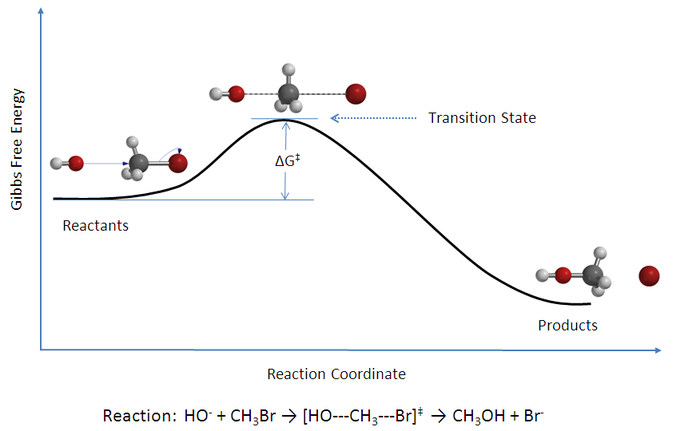Transition state theory: The activated complex, which a kind of reactant-product hybrid, exists at the peak of the reaction coordinate, in what is known as the transition state.

### Postulates of Transition State Theory

According to transition state theory, between the state in which molecules exist as reactants and the state in which they exist as products, there is an intermediate state known as the transition state. The species that forms during the transition state is a higher-energy species known as the activated complex. TST postulates three major factors that determine whether or not a reaction will occur. These factors are:

1. The concentration of the activated complex.
2. The rate at which the activated complex breaks apart.
3. The mechanism by which the activated complex breaks apart; it can either be converted into products, or it can “revert” back to reactants.

This third postulate acts as a kind of qualifier for something we have already explored in our discussion on collision theory. According to collision theory, a successful collision is one in which molecules collide with enough energy and with proper orientation, so that reaction will occur. However, according to transition state theory, a successful collision will not necessarily lead to product formation, but only to the formation of the activated complex. Once the activated complex is formed, it can then continue its transformation into products, or it can revert back to reactants.

### Applications in Biochemistry

Transition state theory is most useful in the field of biochemistry, where it is often used to model reactions catalyzed by enzymes in the body. For instance, by knowing the possible transition states that can form in a given reaction, as well as knowing the various activation energies for each transition state, it becomes possible to predict the course of a biochemical reaction, and to determine its reaction rate and rate constant.